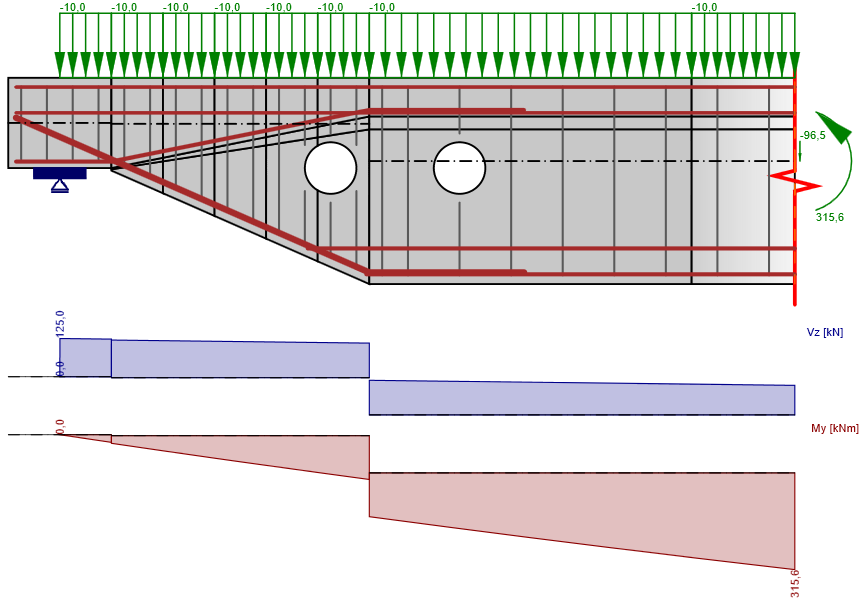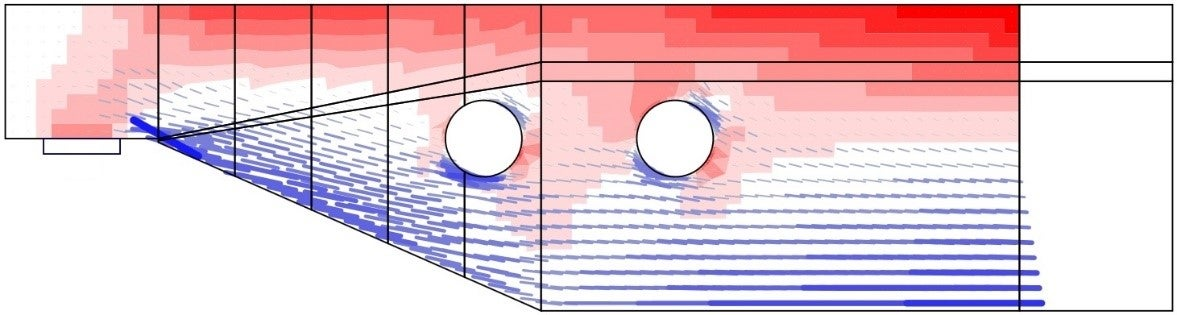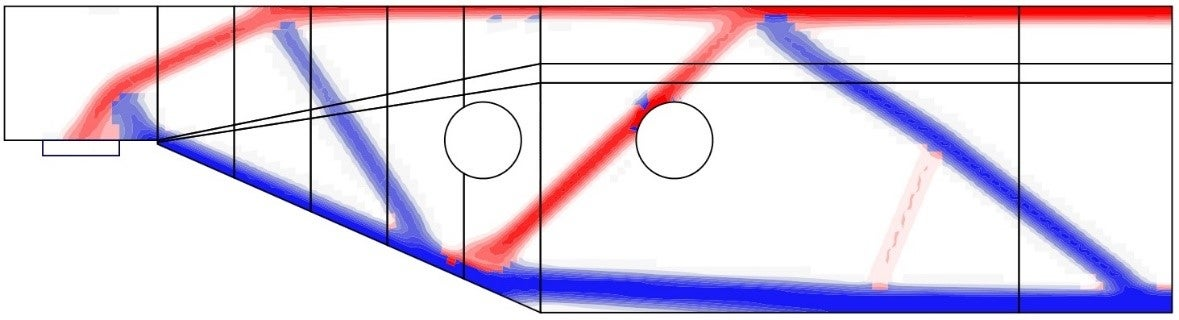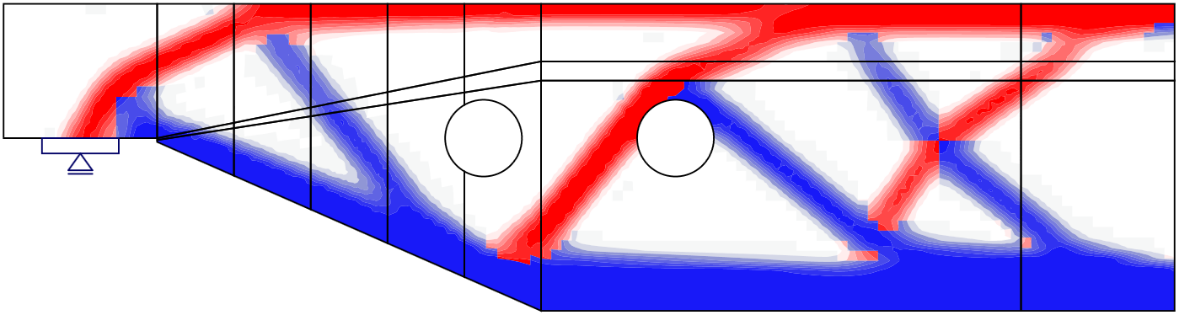# 2 Reinforcement design

Questo articolo è disponibile in:
$$2.1 Workflow and goalsThe goal of reinforcement design tools in the CSFM is to help designers determine the location and required amount of reinforcing bars efficiently. The following tools are available to help/ guide the user in this process: linear calculation, topology optimization and area optimization.Reinforcement design tools consider more simplified constitutive models than the models used for the final verification of the structure. Therefore, the definition of the reinforcement in this step should be considered a pre-design to be confirmed/refined during the final verification step. The use of the different reinforcement design tools will be depicted on the model shown in Fig. 5, which consists of one end of a simply supported beam with variable depth subjected to a uniformly distributed load.$\textsf{\textit{\footnotesize{Fig. 5\qquad Model used to illustrate the use of the reinforcement design tools.}}}$2.2 Reinforcement locationsFor regions where the reinforcement layout is not known beforehand, there are two methods available in the CSFM to help the user determine the optimum location of reinforcing bars: linear analysis and topology optimization. Both tools provide an overview of the location of tensile forces in the uncracked region for a certain load case.2.2.1 Linear analysisLinear analysis considers linear elastic material properties and neglects reinforcement in the concrete region. It is, therefore, a very fast calculation that provides a first insight into the locations of tension and compression areas. An example of such a calculation is shown in Fig. 6.$\textsf{\textit{\footnotesize{Fig. 6\qquad Results from the linear analysis tool for defining reinforcement layout}}}$$\textsf{\textit{\footnotesize{(red: areas in compression, blue: areas in tension).}}}$2.2.2 Topology optimizationTopology optimization is a method that aims to find the optimal distribution of material in a given volume for a certain load configuration. The topology optimization implemented in Idea StatiCa Detail uses a linear finite element model. Each finite element may have a relative density from 0 to 100 %, representing the relative amount of material used. These element densities are the optimization parameters in the optimization problem. The resulting material distribution is considered optimal for the given set of loads if it minimizes the total strain energy of the system. By definition, the optimal distribution is also the geometry that has the largest possible stiffness for the given loads.The iterative optimization process starts with a homogeneous density distribution. The calculation is performed for multiple total volume fractions (20%, 40%, 60% and 80%), which allows the user to select the most practical result, as proposed by . The resulting shape consists of trusses with struts and ties and represents the optimum shape for the given load cases (Fig. 7).$\textsf{\textit{\footnotesize{Fig. 7\qquad Results from the topology optimization design tool with 20\% and 40\% effective volume}}}$$\textsf{\textit{\footnotesize{(red: areas in compression, blue: areas in tension).}}}$$$